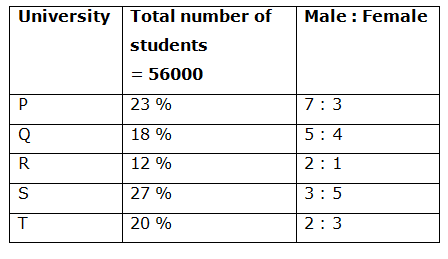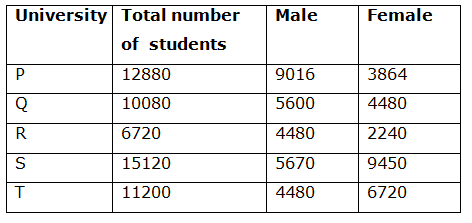# LIC AAO/SBI PO Prelims Quantitative Aptitude Questions 2019 (Day-01)

Dear Aspirants, Our IBPS Guide team is providing new series of Quantitative Aptitude Questions for LIC AAO/SBI PO 2019 so the aspirants can practice it on a daily basis. These questions are framed by our skilled experts after understanding your needs thoroughly. Aspirants can practice these new series questions daily to familiarize with the exact exam pattern and make your preparation effective.

[WpProQuiz 5465]

### Click Here for SBI PO Pre 2019 High-Quality Mocks Exactly on SBI Standard

Directions (1 – 5): Each question below contains a statement followed by Quantity I and Quantity II. Find the relationship among them. Mark your answer accordingly.

a) Quantity I > Quantity II

b) Quantity I < Quantity II

c) Quantity I ≥ Quantity II

d) Quantity I ≤ Quantity II

e) Quantity I = Quantity II (or) relationship cannot be determined

1)

Quantity I: Simple interest earned on a certain sum of money after 4 years at the rate of 8 % per annum is Rs. 17920. Then find the principle amount

Quantity II: Rs.45000

2)

Quantity I: 2/5 minutes

Quantity II: A train can crosses a tree in 8 sec and the same train crosses the platform of length 150 m in 18 sec. Then find the time taken by the bridge of length 210 m?

3)

Quantity I: The area of rectangular garden is 2420 Sq m. The length of the rectangular garden is 25 % more than the breadth. Find the perimeter of the garden

Quantity II: The area of a square park is 2304 Sq m. Find the perimeter of the park

4) A bag contains, 5 pink, 6 yellow and 3 white balls.

Quantity I: If 3 balls are drawn randomly, then find the probability of getting at least one yellow ball?

Quantity II: 9/13

5)

Quantity I: Raji can swim at 8 km/hr in still water. The river flows at 3 km/hr and it takes 6 hours more upstream than downstream for the same distance. How far is the place?

Quantity II: 40 km

Directions (Q. 6 – 10): Study the following information carefully and answer the given questions:

The following table shows the percentage distribution of total number of students who completed their graduation from different universities and the ratio of male to female among them.6) Find the total number of male graduates from the given universities together?

a) 29246

b) 25782

c) 33567

d) 37895

e) None of these

7) Total number of female graduates from university Q and R is approximately what percentage of total number of male graduates from university P and T?

a) 25 %

b) 40 %

c) 70 %

d) 50 %

e) 35 %

8) Find the ratio between the total number of male graduates from university Q, R and T together to that of female graduates from university P, R and S together?

a) 8576: 7115

b) 9254: 8471

c) 7280: 7777

d) 5890: 4783

e) None of these

9) Total number of graduates from university P and Q together is approximately what percentage more/less than the total number of graduates from university R and S together?

a) 5 % less

b) 5 % more

c) 15 % more

d) 15 % less

e) 30 % more

10) In which university has the highest number of female graduates?

a) University P

b) University Q

c) University R

d) University S

e) University T

Direction (1-5) :

Quantity I:

SI = PNR/100

17920 = (P*4*8)/100

P = (17920*100)/32 = Rs. 56000

Quantity II: Rs. 45000

Hence, Quantity I > Quantity II

Quantity I:

2/5 minutes = 2/5 * 60 = 24 sec

Quantity II:

Let the length of train be x,

Speed is equal. So,

x/8 = (x + 150)/18

9x = 4x + 600

5x = 600

X = 120

The length of train = 120 m

Speed of train = 120/8 = 15 m/s

Required time = (120 + 210)/15 = 330/15 = 22 sec

Hence, Quantity I > Quantity II

Quantity I:

The area of rectangular garden = 2080 Sq m

l/b = 5/4 = > l : b = 5 : 4

5x*4x = 2420

20x2 = 2420

X2 = (2420/20) = 121

X = 11

Length = 55 m, Breadth = 44 m

Perimeter of the garden = 2*(l + b) = 2*(55 + 44) =2*99 = 198 m

Quantity II:

The area of a square park = 2304 Sq m

Area (a2) = 2304

Side (a) = 48

Perimeter of the park = 4a = 4*48 = 192 m

Hence, Quantity I > Quantity II

Total number of balls = 5 + 6 + 3 = 14 balls

Quantity I:

n(S) = 14C3 = (14*13*12)/(1*2*3)

The probability of getting at least one yellow ball = 1 – P (None is yellow ball)

P (None is yellow ball)

n(E) = 8C3 = (8*7*6)/(1*2*3)

P(E) = n(E)/n(S) = 8C3 /14C3

= > [(8*7*6)/(1*2*3)] / [(14*13*12)/(1*2*3)]

= > 2/13

Required probability = 1 – (2/13) = 11/13

Quantity II: 9/13

Hence, Quantity I > Quantity II

Quantity I:

x/(8 – 3) – x/(8 + 3) = 6

x/5 – x/11 = 6

6x/55 = 6

X= 55 km

Quantity II: 40 km

Hence, Quantity I > Quantity II

Directions (6 – 10):The total number of male graduates from the given universities together

= > 9016 + 5600 + 4480 + 5670 + 4480

= > 29246

Total number of female graduates from university Q and R

= > 4480 + 2240 = 6720

Total number of male graduates from university P and T

= > 9016 + 4480 = 13496

Required % = (6720/13496)*100 = 49.79 % = 50 %

The total number of male graduates from university Q, R and T together

= > 5600 + 4480 + 4480 = 14560

The total number of female graduates from university P, R and S together

= > 3864 + 2240 + 9450 = 15554

Required ratio = 14560: 15554 = 7280: 7777

Required % = {[(23 + 18) – (12 + 27)]/(12 + 27)}*100

= > {(41 – 39)/39}*100 = 5.128 % = 5 % more